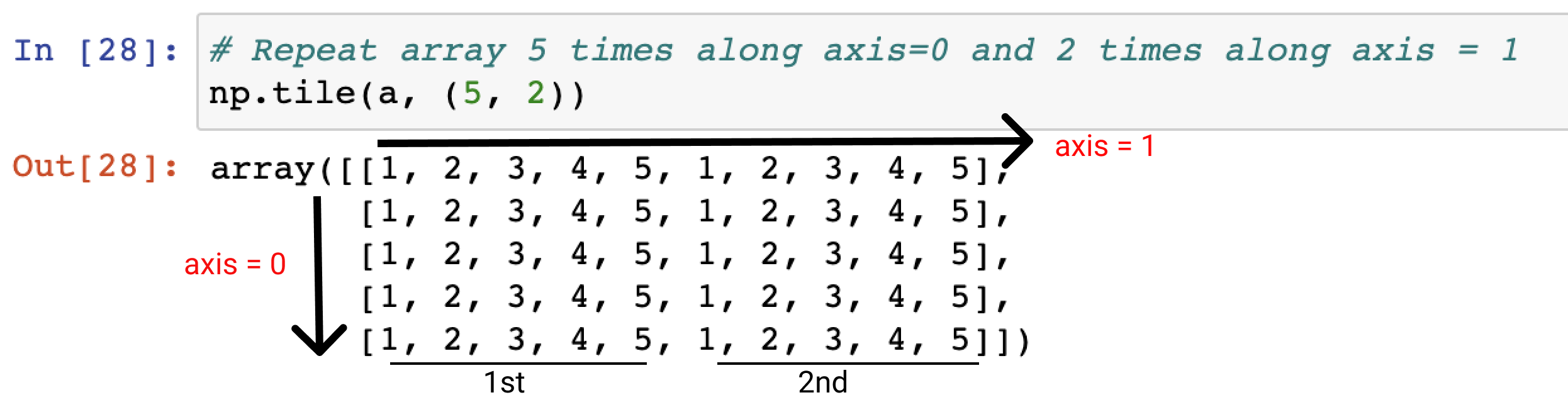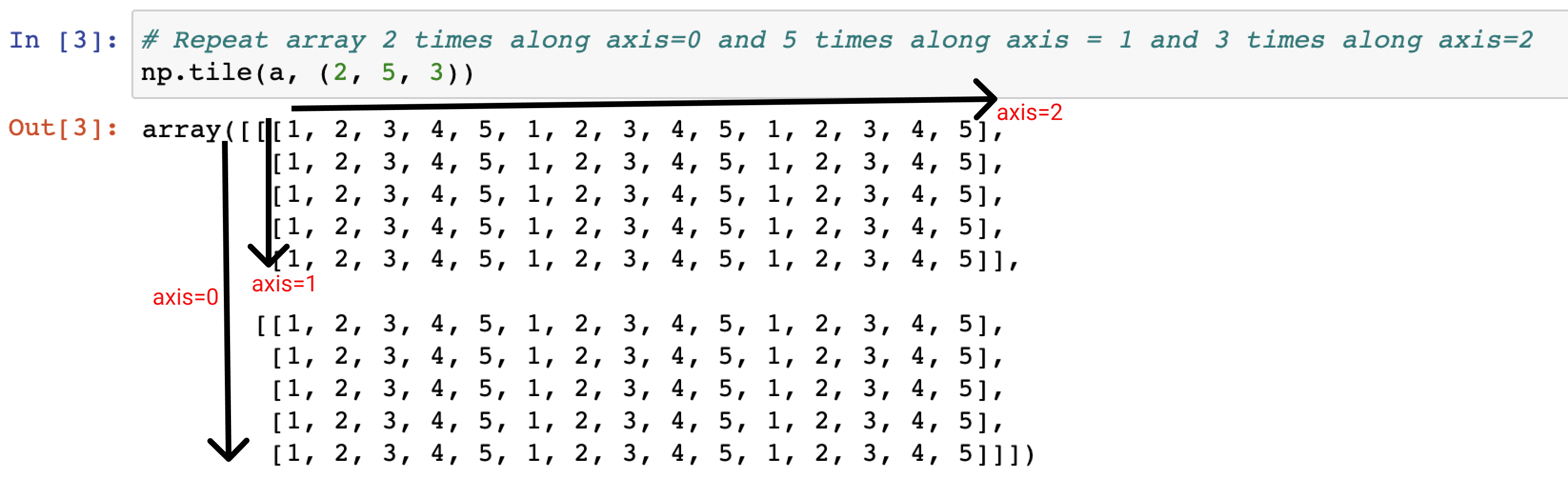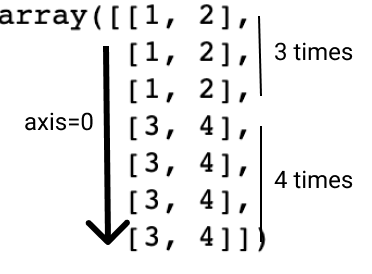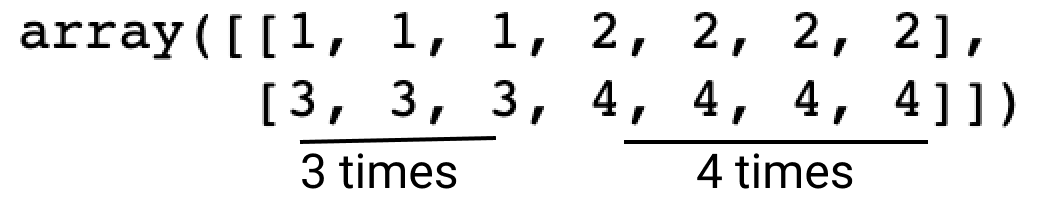# numpy tile vs repeat

In this post, we will learn what is numpy tile and what’s the difference between numpy tiles and repeat

## Numpy tile - numpy.tile()

The numpy tile function is used for repeating an array by any number of times in any dimension of the array

Here is the formula for using numpy tile:

``````numpy.tile(A, reps)
``````

It repeats the array A by number of times given by reps parameter. If reps has length d, the result will have dimension of max(d, A.ndim). Let’s understand this with the help of examples below

### Using Numpy tile in one dimension array

Let’s create a 1D array and we want to repeat this array two times along the axis=0

``````import numpy as np
A = np.array([1, 2, 3, 4, 5])
``````

Pass the reps parameter as 2 and check the result

``````np.tile(A, 2)

# Out
array([1, 2, 3, 4, 5, 1, 2, 3, 4, 5])

``````

So you can see here the output is a 1D array and the elements of original array is repeated twice

### Using Numpy tile to build a 2D array

Let’s use the same 1D array and we will build a 2D array out of it by repeating this array(A), reps param in tile function is given as (5,2) which means repeat the array 5 times along axis=0 and 2 times along the axis=1

``````np.tile(A, (5, 2))
``````

Output:In the above output you can see the initial array(A) is repeated 5 times along the axis=0 and the array is repeated 2 times along the axis=1

### Using Numpy tile to build a 3D array

Let’s use the same 1D array and we will build a 3D array out of it by repeating this array(A), reps param in tile function is given as (2, 5, 3) which means repeat the array 2 times along axis=0, 5 times along the axis=1 and 3 times along axis=2

``````np.tile(A, (2, 5, 3))
``````

Output:In the above output you can see the initial array(A) is repeated along each of dimensions as mentioned in the passed reps parameter value

## Numpy repeat - numpy.repeat()

Unlike numpy tile, numpy repeat helps to repeat the elements of an array

Here is the formula for the numpy repeat:

``````np.repeat(a, repeats, axis=None)
``````

where,
a: Input array
repeats: the number of repetitions for each element and broadcasted to fit the shape of the given axis
axis: Along which axis to repeat values

### numpy repeat 1D array

First create a 1D array

``````a = np.array([1, 2, 3, 4, 5])

Output:
array([1, 2, 3, 4, 5])
``````

Repeat each element of the array 4 times

``````np.repeat(a, 4)

Output:
array([1, 1, 1, 1, 2, 2, 2, 2, 3, 3, 3, 3, 4, 4, 4, 4, 5, 5, 5, 5])
``````

### numpy repeat 2D array

first create a 2D array

``````x = np.array([[1,2],[3,4]])

Output:
array([[1, 2],
[3, 4]])
``````

if no axis is mentioned then a flattened array is returned, lets understand this with an example.

``````np.repeat(x, 2)

Output:
array([1, 1, 2, 2, 3, 3, 4, 4])
``````

Let’s repeat the element of array(x) along the axis 1, 2 times. we will pass the axis parameter value as 1

``````np.repeat(x, 2, axis =1 )

Output:
array([[1, 1, 2, 2],
[3, 3, 4, 4]])
``````

So you can see, each element is repeated 2 times along the axis=1 in the 2D array(x)

### numpy repeat elements of 2D array along axis=0 and axis=1

we will repeat the elements of a 2D array(x) along the axis=0 and pass the repeats parameter as [3, 4] which means repeate the first array 3 times and 2nd array 4 times

``````np.repeat(x, [3, 4], axis=0)
``````Next, we will repeat the elements of a 2D array(x) along the axis=1 and pass the repeats parameter as [3, 4] which means repeate the first elements in the array 3 times and 2nd element in array 4 times

``````np.repeat(x, [3, 4], axis=0)
``````Tags:

Categories:

Updated: